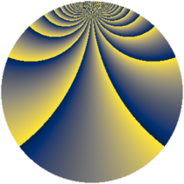# Properties

 Label 2880.2.fnLevel $2880$ Weight $2$ Character orbit 2880.fn Rep. character $\chi_{2880}(77,\cdot)$ Character field $\Q(\zeta_{48})$ Dimension $9152$ Sturm bound $1152$

# Learn more about

## Defining parameters

 Level: $$N$$ $$=$$ $$2880 = 2^{6} \cdot 3^{2} \cdot 5$$ Weight: $$k$$ $$=$$ $$2$$ Character orbit: $$[\chi]$$ $$=$$ 2880.fn (of order $$48$$ and degree $$16$$) Character conductor: $$\operatorname{cond}(\chi)$$ $$=$$ $$2880$$ Character field: $$\Q(\zeta_{48})$$ Sturm bound: $$1152$$

## Dimensions

The following table gives the dimensions of various subspaces of $$M_{2}(2880, [\chi])$$.

Total New Old
Modular forms 9280 9280 0
Cusp forms 9152 9152 0
Eisenstein series 128 128 0

## Trace form

 $$9152q - 24q^{2} - 16q^{3} - 24q^{5} - 32q^{6} - 8q^{7} + O(q^{10})$$ $$9152q - 24q^{2} - 16q^{3} - 24q^{5} - 32q^{6} - 8q^{7} - 32q^{10} - 48q^{11} - 16q^{12} - 8q^{13} - 16q^{15} - 16q^{16} - 16q^{18} - 24q^{20} - 32q^{21} - 8q^{22} - 24q^{23} - 8q^{25} - 16q^{27} - 32q^{28} - 112q^{30} - 24q^{32} - 32q^{34} - 32q^{36} - 32q^{37} - 24q^{38} - 8q^{40} - 48q^{41} - 16q^{42} - 8q^{43} - 16q^{45} - 64q^{46} - 120q^{48} - 336q^{50} - 32q^{51} - 8q^{52} - 32q^{55} - 48q^{56} - 16q^{57} - 8q^{58} + 40q^{60} - 16q^{61} - 32q^{63} - 48q^{65} - 32q^{66} - 8q^{67} - 120q^{68} - 8q^{70} - 136q^{72} - 32q^{73} - 16q^{75} + 32q^{76} - 24q^{77} - 168q^{78} - 32q^{81} - 32q^{82} - 24q^{83} - 48q^{85} - 48q^{86} + 96q^{87} - 8q^{88} - 16q^{90} - 64q^{91} + 432q^{92} - 40q^{93} + 64q^{94} - 32q^{96} + 128q^{99} + O(q^{100})$$

## Decomposition of $$S_{2}^{\mathrm{new}}(2880, [\chi])$$ into newform subspaces

The newforms in this space have not yet been added to the LMFDB.